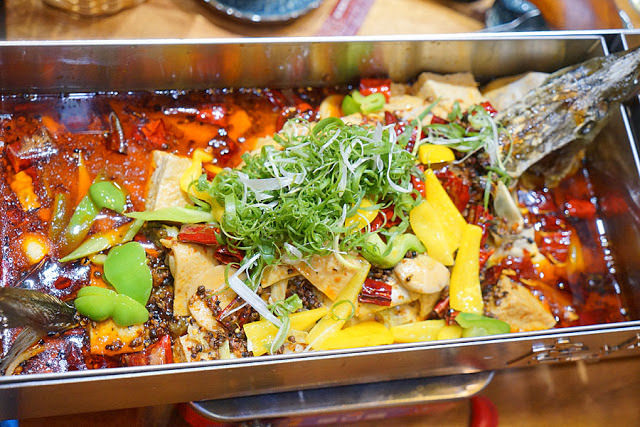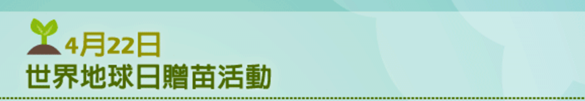## 大同 TATUNG 同樂會 | 大同 3C | 黃金週酬賓盛典 | 大同電烤箱@大同TATUNG同樂會

#大同3C #黃金週酬賓盛典

Q地地的滷豬腳

# 防非洲豬瘟別再說不知道20萬元台幣可是要賺好久的說

PS. 提醒大家收到來路不明的肉品不可以丟廚餘或垃圾桶，請打0800-039-131交專門機構處理。快來加入「台灣旅食」line生活圈，掌握台灣美麗好食光

# 部落客一起來呼應

## 台中烤魚餐廳│營業到凌晨兩點的店小二紅樓夢椒麻烤魚，沒那個屁股就不要點到大辣(新聞稿)2017年的暑假，是台中烤魚餐廳的主市場，短短幾月

## 106年世界地球日 中油公司與您一同守護台灣## 春節尾聲-墾丁之旅二- 墾丁夏都沙灘酒店[海角七號拍攝飯店]

[拍攝海角七號飯店]前，小朋友一直念念不忘的學習騎馬馬場先到了
，也許是過完年了，所以馬場上顯得冷冷清清。DIY的活動[每天課程不一樣]…好多活動等，可以讓小朋友一整天，可是拔拔說那個長度怕車子放不下，這些都是我在網路上找到的。，夏都沙灘酒店在館區內設置寄居蟹生態觀賞區。## 表情符號／顏文字 .

＜（－︿－）＞　我很生氣喔！！別吵我，知道嗎
＜（＞＿＜)  ＞   好
＜（￣︶￣）＞　滿足且得意，得意快樂
＜（￣︶￣）／　滿足並且伸出手，表示贊同、贊成
＜（＠￣︶￣＠）＞　滿足地臉紅
（＃￣▽￣＃）　害羞
＼（￣︶￣）／　抱抱、開心
ｂ（￣▽￣）ｄ　豎起雙手拇指說好
╰（￣▽￣）╭　眉飛色舞
（￣︶￣）↗　出發吧
○（＊￣︶￣＊）○　吃飽睡好
♀（￣▽￣）／　優質女孩
♂（￣▽￣）／　優質男孩
╮（╯◇╰）╭　啾～啾～親一個愛你
╭（′▽‵）╭（′▽‵）╭（′▽‵）╯　GO!
（￣▽￣）～■□～（￣▽￣）　乾杯
＜（￣︶￣）＞＜（￣︶￣）＞＜（￣︶￣）＞　當我們同在一起

（╯‵□′）╯︵┴─┴    翻桌B

╭∩╮（￣▽￣）╭∩╮    哼，去你的

（╬￣皿￣）＝○＃（￣＃）３￣）看拳

＜（‵□′）＞───Ｃε（┬＿┬）３ 　生氣扭耳朵

＜( ￣︿￣)︵θ︵θ︵θ︵θ︵☆（＞口＜－）　無影腳攻擊

＝　＝＃        生氣（青筋暴露）

(#‵′)     哼！

（￣ c￣）y▂ξ 　抽煙

(￣(工)￣) 大狗熊

( ▔___▔)y-～ 抽煙

（～￣▽￣～） 裝傻

╭(─╴╴─)╮ ▃▇ 嗯嗯中別打擾

<(￣3￣)>　嗯嗯中別打擾

－(>口＜-)　嗯嗯中別打擾

<(‵▽′)>   哈哈哈~~(我是壞人~)

o(〒﹏〒)o 淚

╮(￣▽￣”)╭  無奈

ψ(._. )>  作筆記

≧ω≦　可愛、可愛

O口O!　超驚訝!

ο-_-)０)。０)      給你一拳~~
m(_ _)m 　　　　　　萬事拜託(跪地，嗑頭)
(/_) 　　　　　 　　看不到~眼睛被手摭住了
|(- _-)|            沒聽到~耳朵遮住了
(⊙０⊙)            目瞪口呆
⊙ . ⊙   睜大眼
\(^0^)/    舉手歡呼
－ －||||    無奈
?~?    疑問、疑問
◎−◎？   疑問
(T_T)    傷心、我哭了
ＱＱ   流淚
Ｑ０Ｑ    流淚、張大嘴
〒△〒    哭
T△T     哭訴…….
U／／／U     臉紅紅害羞
≧◇≦   感動~
╮(－_－)╭ 兩手一攤~

＝　＝＃ 生氣（青筋暴露）
= 3 = 嘟嘴
( (－_－)y–~~ 抽煙……
(/‵Ⅲ′)/ 抓你來咬!!!
(Q o Q) b 人家才沒有
*(^_^)/* 拿彩球、為你加油~
(#‵′) 啍!!
(>_<) 小生氣
_ /# 我生氣了(横眉豎眼)
(*^．^*) 親一個!!!
(@^０^@)/★ 晚安~~
(>﹏<) 不!!!!!!!!!!!
(*+﹏+*)~@ 受不了~受不了
‵(*∩_∩*)′ 獻上最可愛的笑容
(×_×) 昏倒
……..(><)/ 哇!!出現了!!
=  =b 冒冷汗
^ ^” 笑笑的無奈
(－_－)ｚＺ 睡著了啦～
－▽－ｙ 耶！ ∪＿∪ 恩恩～
((。(^_^)。)) 期待、期待
<(‵▽′)> 哈哈哈~~(我是壞人~)
“(/><)/ 阿達~~ (>c<) 唉唉叫~
(^^;;;;; 緊張、緊張~~
(╯︵╰) 一臉苦瓜
p(^_^)q 加油~(兩手緊握拳高舉)
f(^_^) 這樣啊…
<(“””○”””)> Oh!  My God
(．Q．) 做鬼臉
(*^^)/.。．：*：．。★，。：*。☆ 祝好夢
(“*_*)(‵~~‵)==== 看到鬼，就是看到鬼了嘛
(_ _)(- . -)(~0~) 累了~又瞇眼~又打呵欠的~
(^o^哇)~~(^0^)哈~~(^○^)哈~~ 大笑三聲~~
0(^o^)~~YA(^0^)~~SU(^○^)~~MI(^_^) 好好休息(用於睡前~日文)
～＞＿＜～哭泣中．．．
o_O  訝異
=_=”  無奈
(￣﹁￣)   流口水
( h_n )   大眼睛和小眼睛
‧★,:*:‧(￣▽￣)/‧:*‧°★*   用力灑花恭喜
OrzＯ|￣|＿敗給你了（一個人五體投地的象形符號，有許多衍生變化，例：Orz  Or2

(-ิ_-ิ;)、(-ิo-ิ) 泰國式顏文字
＜（￣︶￣）＞　　　　　滿足且得意，得意快樂
（╬￣皿￣）＝○＃（￣＃）３￣）             看拳
＜（‵□′）＞───Ｃε（┬＿┬）３          生氣扭耳朵
＜( ￣︿￣)︵θ︵θ︵θ︵θ︵☆（＞口＜－）     無影腳攻擊
(((^ ^)(^ ^))) 什麼什麼
(⊙_⊙)<~~啾~~>(#^_^#) 親一個
(^ ^)／▽▽＼(^ ^) 乾杯~~杯子碰杯子
(^_^)／★＼(^_^) 乾杯(杯子相碰)
(^_-)db(-_^) 手指打勾勾~約定!
╭（′▽‵）╭（′▽‵）╭（′▽‵）╯　　　　GO!
（￣▽￣）～■□～（￣▽￣）　　　　　　　　　乾杯
＜（￣︶￣）＞＜（￣︶￣）＞＜（￣︶￣）＞　　當我們同在一起

┌──┐
│  　├╮
│██├╯
╰──╯ 　請你喝咖啡…

○
∣＼)
﹡﹡﹡◎¯≧¯◎　騎機車

／＼／＼
＼　　／
＼／　　愛心

／＼／＼／＼
＜－＼－　／　／－＜＜
＼／＼／　　　　　　　一串心

Ｏ　 \\|// 　 －＝＝　）。只。）　踢飛

╭☆╭★﹒☆╭★﹒☆╭★﹒☆╭★﹒☆╭★│
╱╲*╱╲　★．﹒★╱‧°相信有一天﹒
`╱╱　 ◢◣+　 　╳　　╲　　．
`╱　+ ◢█◣　◢◣ 最亮的那顆星也會落在你身上
▄█▄▆██▆▄█▆▄▄█▄▆██▆▄▄

◤┼。o＋○。 Ｏ‧°o‧°o╯○。o＋○。 Ｏo‧°°o ◥
╔＊═╗ ╔╗　　　 　　╔╗╔╗
╚╗╔╝ ║║★═╦╦╦═╗ ║☆╝╠═╦╦╗
╔╝╚╗ ＊╚╣║║║║╠╣ ╚╗╔╣║♀║☆
╚═♂╝ ╚═╩═╩＊╩═╝   ╚╝╚═╩═╝
◣┼。o＋○。 Ｏ‧°o‧°o╯○。o＋○。 Ｏo‧°°o ◢
(~愛一個人不一定要擁有~但擁有一個人就一定要好好愛他~)

o_O　訝異
◎−◎？　疑問
^_^　高興
T_T　哭得很傷心
=_=”　無奈
=3=　扭計、嘟嘴
\$_\$　見錢眼開
(^_-)db(-_^)　手指打勾勾、約定
= =b　冒冷汗
╮(－_－)╭　兩手一攤
＝　＝＃　生氣（青筋暴露）
|(-_-)|　沒聽到（耳朵遮住了）
/.\　失望
>< 表示傷心、無奈、嗔怒的廣義表現符號
ˊˋ因難過而眉毛倒豎

(=^_^=)　喵喵
((。(^_^)。))　期待、期待
*\(^_^)/*　拿綵球、為你加油
≧◇≦　感動
(^o^)哈~~(^0^)哈~~(^○^)哈~~　大笑3聲
(￣﹁￣)　流口水
( h_n )　大眼睛和小眼睛
‧★,:*:‧\(￣▽￣)/‧:*‧°★*　用力灑花恭喜
v(￣︶￣)y　得意
ㄟ(￣▽￣ㄟ)　偷偷逃跑
( ￣□￣)/ <(￣ㄧ￣ ) <(￣ㄧ￣ ) 致敬

Orz、Ｏ|￣|＿　敗給你了（一個人五體投地的象形符號，有許多衍生變化，例：Orz Or2 srO OTZ 囧rz）
OGC、OG[ （自慰）

^(00)^　小豬
= =+
(˙_˙)/
>//////< 臉紅(害羞或激動)
!_!
@_@
*_*
+_+
@[]@
WoW
vov
^Q^
~.~
>3<　kiss
XD、XP、x)

(*￣▽￣)/‧★*”`’*-.,_,.-*’`”*-.,_☆,.-*`’`*-　流星拳

（┬＿┬）　流淚
（＞﹏＜）　痛苦
（┬＿┬）↘　跌倒
〒▽〒　真命苦
～＞＿＜～　哭泣中
T^T”///　飆淚
>3<　親一個
(T_T)　傷心、我哭了

（︶︿︶）　不滿
╰（‵□′）╯　暴怒
（︶︿︶）＝凸　比中指
（╯－＿－）╯╧╧　翻桌
（╯‵□′）╯︵┴─┴　翻桌
╭∩╮（￣▽￣）╭∩╮　哼，去你的（鄙視你）
（╬￣皿￣）＝○＃（￣＃）３￣）　看拳
＜（‵□′）＞───Ｃε（┬＿┬）３　生氣扭耳朵
＜( ￣︿￣)︵θ︵θ︵θ︵θ︵☆（＞口＜－）　無影腳攻擊
＝　＝＃　生氣（青筋暴露）
(#‵′)　哼！
\(@^０^@)/★　晚安
(^o^哇)~~(^0^)哈~~(^○^)哈~~　大笑三聲
0(^o^)~~YA(^0^)~~SU(^○^)~~MI(^_^) 好好休息（用於睡前、日文）
<*^.^*>　大笑

〈（＿　＿）〉　道歉、鞠躬
ｍ（＿　＿）ｍ　叩拜
(^〈^)　對不起啦

╮（￣▽￣）╭
－ －||||
┐（─__─）┌
╮(︶︿︶)╭

(￣(工)￣)　大狗熊
(*> ω <*)超害羞
( ▔___▔)y-～　抽菸
( ￣ c￣)y▂ξ　抽菸
（～￣▽￣～）　裝傻
╭(─╴╴─)╮ ▃▇　嗯嗯中，別打擾
<(￣3￣)>
－(>口＜-)
<(‵▽′)>　哈哈哈！我是壞人
o(〒﹏〒)o　淚
O-(///￣皿￣)☞ ─═≡☆゜★█▇▆▅▄▃▂＿　佛地魔版之去去武器走
( ☉_☉)≡☞o────★°　哈利波特版之去去武器走
○(￣︿￣) ○─═★°　榮恩版之去去武器走
╮(￣▽￣”)╭　無奈
ψ(._. )>　筆記
≧ω≦　可愛、可愛
O口O!　超驚訝!
(*^．^*)　親一個
(^_-)db(-_^)　手指打勾勾、約定
◢▆▅▄▃崩╰(〒皿〒)╯潰▃▄▅▇◣

||||| 愉快時 |||||

└(^o^)┘; 偶頭好狀壯ㄋㄟ“`
﹌○﹋ 喔嗨呦^ˇ^≡
~^o^~ 大家安安!!
=^_^= 溫馨的微笑!!!
Y(^_^)Y 舉雙手勝利
\^o^/ 歡呼
^o^y 勝利^o^y
↖(^ω^)↗ 小豬為你打氣！
~^o^~ 加油呦!
*^ˍ^* 笑，打
*^ο^* 哦～，呵呵傻笑
*^◎^* 呵呵大笑（嘴唇好厚）
*^÷^* 得意的笑（有上下唇的喲）
~~~^_^~~~ 笑斃囉（笑得連眼淚都蹦出來了…）
(-.-)=3 鬆ㄌ一口氣~
~~~///(^v^)\\\~~~ 微笑表示友善!!!~哈~哈~
~@^_^@~ 可愛呦!
\\*^o^*// 可愛ㄋㄟ~
~*.*~ 害羞又迷人的小女生
#^_^# 臉紅了!!
∩__∩y 耶~~^^ (裝可愛?!)
（*^＠^*） 乖～（還含個奶嘴哦）

||||| 不愉快時 |||||

X﹏X 糟糕．． 完蛋的意思呀~~
(° ο°)~@ 暈倒了..
{{{(>_<)}}} 發抖
╯﹏╰ 粉無奈~~
\(╯-╰)/ 很沒勁/無耐的意思
（╯︿╰﹀ 一臉苦瓜
︸_︸ 粉無奈..粉悲情
-____-” 唉~~別提了…..
。_。 受到打擊，表情呈現呆滯樣～
（*@︿@*﹀ 悲，暈
-︵- 好傷心.
//(ㄒoㄒ)// 流淚中…
::>_<:: 哭
〒_〒 鳴~~我在哭…
%>_<% 我要哭了哦…
╰_╯ 我發火了!! 生氣的意思~~~眉毛都翹起來~~
>_<# 粉生氣~冒青筋
@x@ 生氣
（ˋ︿ˊ﹀-# 生氣
（＞﹏＜） 不～
（*+﹏+*）~ @ 受不了～
x__x, 唉….. 別哭了!

||||| 狀況外時 |||||

>_<||| 很尷尬~!!!
^_^; 尷尬
⊙﹏⊙∥∣° 真尷尬～～
^_^||| 好尷尬！
^_^” 尷尬的笑…..
→_→ 懷疑的眼神~~
..@_@|||||.. 頭昏眼花
…(⊙_⊙;)… ○圭~○列~~怎麼醬？
o_o …. 沉思(說穿了”呆滯狀況”)
O__O” 呆滯的眼神~~~!!
///^_^……. 造成”冷”…乾笑~
?o?||| 聽無(就是聽不懂ㄉ意思啦^^)
( ^_^ )? 什麼意思??
(+_+)? 不知道你在說什麼
（?ε?）? 哩公啥哇聽無啦~
o_O??? 發生啥事?
@_@a 搔頭，疑惑

>”<|||| 傷腦筋的意思~

||||| 訝異 |||||

‵(*>﹏<*)′ 好刺激..
（*@ο@*） 哇～
O_o 驚訝~~~
（⊙ｏ⊙） 目瞪口呆
（°ο°）~ @ 暈倒，不省人事
*@_@* 崇拜的眼神;眼睛為之一亮
★~★ 見到偶像眼睛為之一亮

||||| 示愛 |||||

^◎- 愛你呦~~
(*^‧^*) 啵一個!
(^_^)∠※ 送妳一束花
（*^﹏^*）′超級羞羞
（*^︹^*︺ 羞羞
（*∩_∩*）獻上

||||| 愛睏 |||||

~_~ 想睡覺了的意思!!
(～ o ～)~zZ 我想睡啦～
π_π 打瞌睡

||||| 感動 |||||

~>_<~+ 好感人唷…
Q_Q 流眼淚的樣子!!
（＋﹏＋）流淚，感動
（＋﹏＋）超級感動，眼淚不停
^_^o ~~~ 感動感動~~~

||||| 其他 |||||

=@~@= 一個看到美眉就緊張+臉紅的大學生。
@(一-一)“““`秘密——–噓
（ˇ︿ˇ﹀ 不以為然
(^人^) 拜託啦^^~
(^_^)/~~ 掰掰~
*x*… … 你逼我也沒用,我劫對不會搜粗棄的!
~w_w~ … … 噓!別吵偶,讓偶思考一下!
（ˇˍˇ） 嗯～，思考中
<{=．．． 烏鴉烏鴉飛過去表示冷場,無趣…..
（*>.<*）~ @ 酸～
~`o`~ 了解
ˋ_ˊ* 你不要命啦!!!
-.- 裝蒜

╭(　▔▽▔)╮我今天要表演變章魚！
╜　╙

╭(　▔▽▔)╯要開始摟～
╜　╙

╰(　≧﹏≦)╯嗚阿。。
╜　╙

碰！！！

(　▔◎▔) 喔耶～變身完畢！
╱│││╲

(‖▔◎▔) 　ㄜ。。
╱│││╲

(‖╤◎╤) 　阿勒～變不回來了
╱│││╲

||||| 精選 |||||

└(^o^)┘; 偶頭好狀壯ㄋㄟ“`
﹌○﹋ 喔嗨呦^ˇ^≡
~^o^~ 大家安安!!
=^_^= 溫馨的微笑!!!
Y(^_^)Y 舉雙手勝利
\^o^/ 歡呼
^o^y 勝利^o^y
↖(^ω^)↗ 小豬為你打氣！
~^o^~ 加油呦!
*^ˍ^* 笑，打
*^ο^* 哦～，呵呵傻笑
*^◎^* 呵呵大笑（嘴唇好厚）
*^÷^* 得意的笑（有上下唇的喲）
~~~^_^~~~ 笑斃囉（笑得連眼淚都蹦出來了…）
(-.-)=3 鬆ㄌ一口氣~
~~~///(^v^)\\\~~~ 微笑表示友善!!!~哈~哈~
~@^_^@~ 可愛呦!
\\*^o^*// 可愛ㄋㄟ~
~*.*~ 害羞又迷人的小女生
#^_^# 臉紅了!!
∩__∩y 耶~~^^ (裝可愛?!)
（*^＠^*） 乖～（還含個奶嘴哦）
(° ο°)~@ 暈倒了..
{{{(>_<)}}} 發抖
╯﹏╰ 粉無奈~~
\(╯-╰)/ 很沒勁/無耐的意思
（╯︿╰﹀ 一臉苦瓜
︸_︸ 粉無奈..粉悲情
-____-” 唉~~別提了…..
。_。 受到打擊，表情呈現呆滯樣～
（*@︿@*﹀ 悲，暈
-︵- 好傷心.
//(ㄒoㄒ)// 流淚中…
::>_<:: 哭
〒_〒 鳴~~我在哭…
%>_<% 我要哭了哦…
x__x, 唉….. 別哭了!
>_<# 粉生氣~冒青筋
@x@ 生氣
（ˋ︿ˊ﹀-# 生氣
（＞﹏＜） 不～
（*+﹏+*）~ @ 受不了～
╰_╯我發火了!! 生氣的意思~~~眉毛都翹起來~~
X﹏X糟糕．． 完蛋的意思呀
>_<||| 很尷尬~!!!
^_^; 尷尬
⊙﹏⊙∥∣° 真尷尬～～
^_^||| 好尷尬！
^_^” 尷尬的笑…..
→_→ 懷疑的眼神~~
..@_@|||||.. 頭昏眼花
…(⊙_⊙;)… ○圭~○列~~怎麼醬？
o_o …. 沉思(說穿了”呆滯狀況”)
O__O” 呆滯的眼神~~